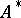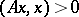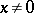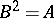##### Actions

(diff) ← Older revision | Latest revision (diff) | Newer revision → (diff)
A linear transformation of a Euclidean or unitary space that coincides with its adjoint linear transformation. A self-adjoint linear transformation in a Euclidean space is also called symmetric, and in a unitary space, Hermitian. A necessary and sufficient condition for the self-adjointness of a linear transformation of a finite-dimensional space is that its matrixin an arbitrary orthonormal basis coincides with the adjoint matrix, that is, it is a symmetric matrix (in the Euclidean case), or a Hermitian matrix (in the unitary case). The eigenvalues of a self-adjoint linear transformation are real (even in the unitary case), and the eigenvectors corresponding to different eigenvalues are orthogonal. A linear transformation of a finite-dimensional spaceis self-adjoint if and only ifhas an orthonormal basis consisting of eigenvectors; in this basis the transformation can be described by a real diagonal matrix.
A self-adjoint linear transformationis non-negative (or positive semi-definite) iffor any vector, and positive definite iffor any. For a self-adjoint linear transformation in a finite-dimensional space to be non-negative (respectively, positive-definite) it is necessary and sufficient that all its eigenvalues are non-negative (respectively, positive), or that the corresponding matrix is positive semi-definite (respectively, positive-definite). In this case there is a unique non-negative self-adjoint linear transformationsatisfying the condition, that is,is the square root of the self-adjoint linear transformation.